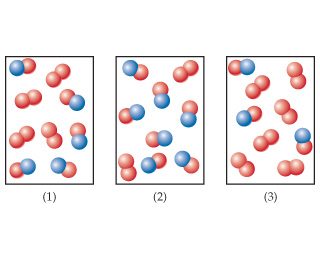Chemistry Practice Problems Reaction Mechanism Practice Problems Solution: The following diagrams represent mixtures of NO(g)...

⚠️Our tutors found the solution shown to be helpful for the problem you're searching for. We don't have the exact solution yet.

# Solution: The following diagrams represent mixtures of NO(g) and O2(g). These two substances react as follows:2 NO ( g ) + O2 ( g ) → 2 NO2 ( g )It has been determined experimentally that the rate is second order in NO and first order in O2.Based on this fact, which of the following mixtures will have the fastest initial rate?

###### Problem

The following diagrams represent mixtures of NO(g) and O2(g). These two substances react as follows:
2 NO ( g ) + O2 ( g ) → 2 NO2 ( g )
It has been determined experimentally that the rate is second order in NO and first order in O2.

Based on this fact, which of the following mixtures will have the fastest initial rate?Reaction Mechanism

Reaction Mechanism

#### Q. Consider the diagram below, which represents two steps in an overall reaction. The red spheres are oxygen, the blue ones nitrogen, and the green ones ...

Solved • Thu Dec 06 2018 09:35:36 GMT-0500 (EST)

Reaction Mechanism

#### Q. Consider the diagram below, which represents two steps in an overall reaction. The red spheres are oxygen, the blue ones nitrogen, and the green ones ...

Solved • Thu Dec 06 2018 09:35:36 GMT-0500 (EST)

Reaction Mechanism

#### Q. What is the molecularity of each of the following elementary reactions? Write the rate law for the reaction.Cl2 (g) → 2 Cl (g)

Solved • Thu Dec 06 2018 09:35:36 GMT-0500 (EST)

Reaction Mechanism

#### Q. What is the molecularity of each of the following elementary reactions? Write the rate law for the reaction.OCl- (aq) + H2O (l) → HOCl (aq) + OH- (aq)...

Solved • Thu Dec 06 2018 09:35:36 GMT-0500 (EST)